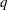The CATMOD Procedure
 REPEATED Statement
REPEATED factor-description<, ..., factor-description></ options> ;

where a factor-description is defined as follows:

factor-name <\$><levels>

and factor-descriptions are separated from each other by a comma. The \$ is required for character-valued factors. The value of levels provides the number of levels of the repeated measurement factor identified by a given factor-name. For only one repeated measurement factor, levels is optional; for two or more repeated measurement factors, it is required. The REPEATED statement incorporates repeated measurement factors into the model. You can use this statement whenever there is more than one dependent variable and the keyword _RESPONSE_ is specified in the MODEL statement. If the dependent variables correspond to one or more repeated measurement factors, you can use the REPEATED statement to define _RESPONSE_ in terms of those factors. You can specify the name, type, and number of levels of each factor, as well as the identification of each level.

You cannot specify the REPEATED statement for an analysis that also contains the FACTORS or LOGLIN statement since all of them specify the same information: how to partition the variation among the response functions within a population.

You can specify the following terms in the REPEATED statement:

factor-name

names a repeated measurement factor that corresponds to two or more response functions. This name must be a valid SAS variable name, and it should not be the same as the name of a variable that already exists in the data set being analyzed.

\$

indicates that the factor is character-valued. If the \$ is omitted, then the CATMOD procedure assumes that the factor is numeric. The type of the factor is relevant only when you use the PROFILE= option or when the _RESPONSE_= option specifies nested-by-value effects.

levels

specifies the number of levels of the corresponding repeated measurement factor. If there is only one such factor and the number is omitted, then PROC CATMOD assumes that the number of levels is equal to the number of response functions per population (). Unless you specify the PROFILE= option, the numbermust either be equal to or be a multiple of the product of the number of levels of all the factors.

You can specify the following options in the REPEATED statement after a slash.

PROFILE=(matrix)

specifies the values assumed by the factors for each response function. There should be one column for each factor, and the values in a given column should match the type (character or numeric) of the corresponding factor. Character values are restricted to 16 characters or less. If there areresponse functions per population, then the matrix must haverows, wheremust either be equal to or be a multiple of. Adjacent rows of the matrix should be separated by a comma.

The values in the PROFILE matrix are useful for specifying models in those situations where the study design is not a full factorial with respect to the factors. They can also be used to specify nested-with-value effects in the _RESPONSE_= option. If you specify character values in both the PROFILE= option and the _RESPONSE_= option, then the values must match with respect to whether or not they are enclosed in quotes (that is, they must be enclosed in quotes in both places or in neither place).

_RESPONSE_=effects

specifies design effects. The variables named in the effects must be factor-names that appear in the REPEATED statement. If the _RESPONSE_= option is omitted, then PROC CATMOD builds a full factorial _RESPONSE_ effect with respect to the repeated measurement factors. For example, the following two statements are equivalent in that they produce the same parameter estimates:

```repeated Time 2, Treatment 2;
repeated Time 2, Treatment 2 / _response_=Time|Treatment;
```

However, the second statement produces tests of the Time, Treatment, and Time*Treatment effects in the "Analysis of Variance" table, whereas the first statement produces a single test for the combined effects in _RESPONSE_.

TITLE=’title’

displays the title at the top of certain pages of output that correspond to this REPEATED statement.

For further information and numerous examples of the REPEATED statement, see the section Repeated Measures Analysis.Previous Page | Next Page | Top of Page